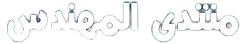# MATHEMATICAL MODELING OF RECTANGULAR LAMINATED PLATES IN BENDING

From the point of view of mechanics of engineering materials,
the deformation of a plate subjected to transverse loading consists of two
components: flexural deformation due to rotation of cross-sections, and shear
deformation due to sliding of sections or layers. The resulting deformation
depends on two parameters: the thickness to length ratio and the ratio of elastic
to shear moduli. When the thickness to length ratio is small, the plate is
considered thin, and it deforms mainly by flexure or bending; whereas when the
thickness to length and the modular ratios are both large, the plate deforms
mainly through shear. Due to the high ratio of in-plane modulus to transverse
shear modulus, the shear deformation effects are more pronounced in the
composite laminates subjected to transverse loads than in the isotropic plates
under similar loading conditions.
Mathematical models for rectangular laminated plates in bending need to
determine the real stress‐strain state in the laminated plate, which requires the
application of more accurate theories. In addition, it is important to find a
balance between the desired accuracy and calculation costs.
Different theories for rectangular plate analysis have been reviewed. These
theories can be divided into two major categories, the individual layer theories
(IL), and the equivalent single layer (ESL) theories. These categories are further
divided into sub – theories by the introduction of different assumptions.
IJBE-9-1-2021_Elmardi_15_34.pdf (540٫0% u)

1 Like